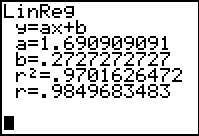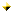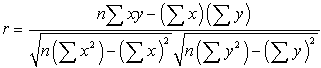Correlation Coefficient

How well does your regression equation truly represent

One of the ways to determine the answer to this question is to
exam the  correlation coefficient and the coefficient of determination.The correlation coefficient, r, and  the coefficient of determination, r 2 , will appear on the screen that shows the regression equation information  (be sure the Diagnostics are turned on --- 2nd Catalog (above 0), arrow down to DiagnosticOn, press ENTER twice.)

 In addition to appearing with the regression information, the values r and r 2 can be found under VARS, #5 Statistics → EQ #7 r and #8 r 2 .

 Correlation Coefficient, r :The quantity r, called the linear correlation coefficient, measures the strength and
the direction of a linear relationship between two variables.
The linear correlation
coefficient is sometimes referred to as the Pearson product moment correlation coefficient in
honor of its developer Karl Pearson.The mathematical formula for computing r is:where n is the number of pairs of data.
(Aren't you glad you have a graphing calculator that computes this formula?)The value of r is such that -1 < r < +1.  The + and – signs are used for positive
linear correlations and negative linear correlations, respectively.Positive correlation:    If x and y have a strong positive linear correlation, r is close
to +1.  An r value of exactly +1 indicates a perfect positive fit.
Positive values
indicate a relationship between x and y variables such that as values for x increases,
values for  y also increase.Negative correlation:   If x and y have a strong negative linear correlation, r is close
to -1.  An r value of exactly -1 indicates a perfect negative fit.
Negative values
indicate a relationship between x and y such that as values for x increase, values
for y decrease.No correlation:  If there is no linear correlation or a weak linear correlation, r is
close to 0.
A value near zero means that there is a random, nonlinear relationship
between the two variablesNote that r is a dimensionless quantity; that is, it does not depend on the units
employed.A perfect correlation of ± 1 occurs only when the data points all lie exactly on a
straight line.  If r = +1, the slope of this line is positive.  If r = -1, the slope of this
line is negative.A correlation greater than 0.8 is generally described as strong, whereas a correlation
less than 0.5 is generally described as weak
These values can vary based upon the
"type" of data being examined.  A study utilizing scientific data may require a stronger
correlation than a study using social science data.

 Coefficient of Determination, r 2  or  R2 :The coefficient of determination, r 2, is useful because it gives the proportion of
the variance (fluctuation) of one variable that is predictable from the other variable
.

It is a measure that allows us to determine how certain one can be in making
predictions from a certain model/graph.The coefficient of determination is the ratio of the explained variation to the total
variation.The coefficient of determination is such that 0 <  r 2 < 1,  and denotes the strength
of the linear association between x and yThe coefficient of determination represents the percent of the data that is the closest
to the line of best fit.  For example, if r = 0.922, then r 2 = 0.850, which means that
85% of the total variation in y can be explained by the linear relationship between x

and y (as described by the regression equation).  The other 15% of the total variation
in y remains unexplained.The coefficient of determination is a measure of how well the regression line
represents the data.  If the regression line passes exactly through every point on the
scatter plot, it would be able to explain all of the variation. The further the line is
away from the points, the less it is able to explain.Finding Your Way Around TABLE of  CONTENTS